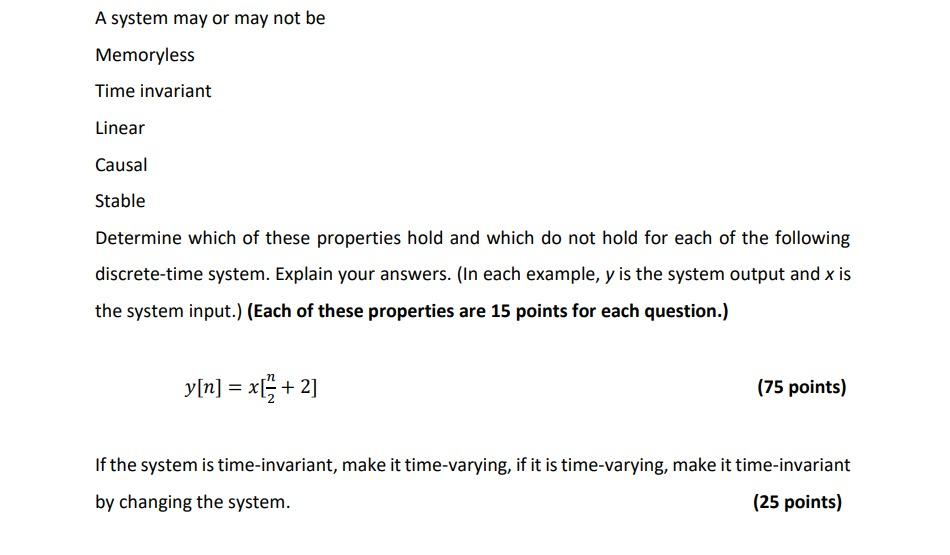# Question A system may or may not be Memoryless Time invariant Linear Causal Stable Determine which of these properties hold and which do not hold for each of the following discrete-time system. Explain your answers. (In each example, y is the system output and x is the system input.) (Each of these properties are 15 points for each question.) y[n] = x + 2] = (75 points) If the system is time-invariant, make it time-varying, if it is time-varying, make it time-invariant by changing the system. (25 points)IKHYUJ The Asker · Computer ScienceTranscribed Image Text: A system may or may not be Memoryless Time invariant Linear Causal Stable Determine which of these properties hold and which do not hold for each of the following discrete-time system. Explain your answers. (In each example, y is the system output and x is the system input.) (Each of these properties are 15 points for each question.) y[n] = x + 2] = (75 points) If the system is time-invariant, make it time-varying, if it is time-varying, make it time-invariant by changing the system. (25 points)
More
Transcribed Image Text: A system may or may not be Memoryless Time invariant Linear Causal Stable Determine which of these properties hold and which do not hold for each of the following discrete-time system. Explain your answers. (In each example, y is the system output and x is the system input.) (Each of these properties are 15 points for each question.) y[n] = x + 2] = (75 points) If the system is time-invariant, make it time-varying, if it is time-varying, make it time-invariant by changing the system. (25 points)